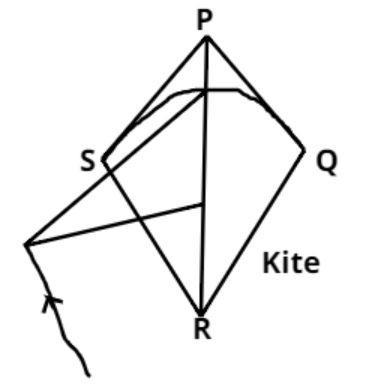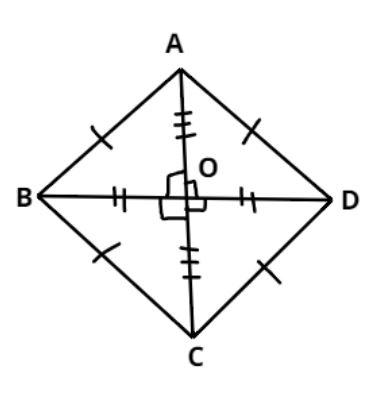QuestionAnswers

# State true or false;All kites are rhombuses.$(a){\text{ True}} \\ (b){\text{ False}} \\ (c){\text{ Ambiguous}} \\ (d){\text{ Data insufficient}} \\$

Hint: Kites are also known as deltoids, deltoid means an unrelated geometric object, however rhombus is a determined geometry and it more looks like a diamond. Use these facts to figure out whether a kite is a rhombus or not.(I.e. OA = OC, OB = OD, $\angle ABC = \angle ADC$ and $\angle BAD = \angle BCD$ )